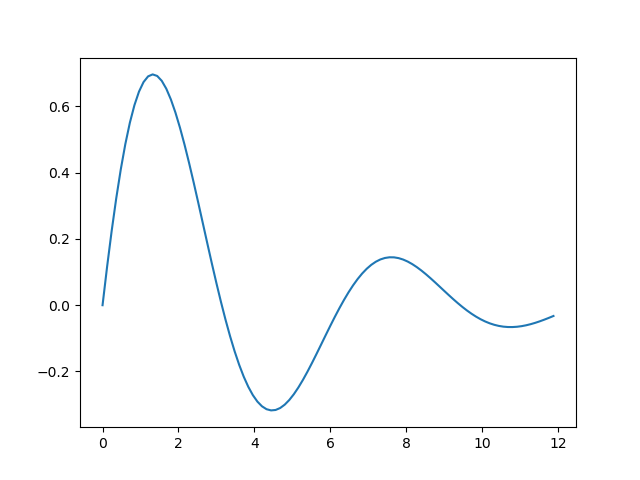# Using numpy with Matplotlib

Martin McBride, 2019-03-03
Tags numeric python linspace function plot pandas matplotlib scipy data science
Categories matplotlib numpyThe previous article showed how to create a plot using Python lists to store the data. Matplotlib also works with numpy, and this can often simplify things.

Here we will look at how to use numpy to create the x values and y values more easily.

## Creating the x values

We want to create a set of 100 x values, equally spaced over the range 0 to 12. We can do this using Python lists by scaling the loop variable.

However, numpy has a function linspace that is designed to do this job. It has two advantages:

• it covers the exact range 0 to 12, with the intermediate values equally spaced

If you recall, our previous code went from 0 to almost 12, which wasn't really much of a problem, but it wasn't quite correct.

Here is how we use linspace to create our range:

xa = np.linspace(0, 12, 100)


## Creating the y values

numpy supports universal functions. These allow you to operate on entire arrays in one go:

ya = np.sin(xa)*np.exp(-xa/4)


This performs the same calculation on each of the 100 elements of xa, creating a new 100 element array ya containing the results. This avoids a loop, which makes the code more readable, but also more efficient.

## Complete code

Here is the complete code:

from matplotlib import pyplot as plt
import numpy as np

xa = np.linspace(0, 12, 100)
ya = np.sin(xa)*np.exp(-xa/4)

plt.plot(xa, ya)
plt.show()


This code is shorter and more readable, as well as being more efficient. This becomes more important with large data sets, and especially 2 dimensional data.

Here is the output, which look exactly the same as before:## Matplotlib for data science

NumPy is a key component used in data science applications, along with Pandas and SciPy. Matplotlib works well with these libraries, making it a very useful library for data science visualisation.

Visit the PythonInformer Discussion Forum for numeric Python.

If you found this article useful, you might be interested in the course Introduction to Matplotlib by the same author.

#### Popular tags

2d arrays abstract data type alignment and angle animation arange arc array arrays behavioural pattern bezier curve built-in function callable object chain circle classes clipping close closure cmyk colour combinations comparison operator comprehension context context manager conversion count creational pattern data science data types design pattern device space dictionary drawing duck typing efficiency ellipse else encryption enumerate fill filter font font style for loop function function composition function plot functools game development generativepy tutorial generator geometry gif global variable gradient greyscale higher order function hsl html image image processing imagesurface immutable object in operator index inner function input installing iter iterable iterator itertools join l system lambda function len line linear gradient linspace list list comprehension logical operator lru_cache magic method mandelbrot mandelbrot set map matplotlib monad mutability named parameter numeric python numpy object open operator optimisation optional parameter or pandas partial application path pattern permutations polygon positional parameter print pure function python standard library radial gradient range recipes rectangle recursion reduce repeat rgb rotation roundrect scaling scipy sector segment sequence setup shape singleton slice slicing sound spirograph sprite square str stream string stroke structural pattern subpath symmetric encryption template text text metrics tinkerbell fractal transform translation transparency triangle truthy value tuple turtle unpacking user space vectorisation webserver website while loop zip